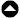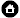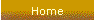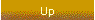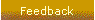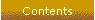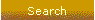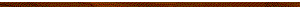## Calibrating Coil System

The Calibrating Coil System (CCS) of LEMI design has four square coil rigidly mounted on massive table and Clarke-Hess instrument set for precision calibration of magnetic field sensors in wide frequency range, see Figs. 1, 2. The calibrating process can be provided both in hand and fully automated mode, with use of specially developed LEMI software and usual desktop or laptop PC. For convenience of tested sensors placement the table surface is displaced below the coils axis at distance 43 mm.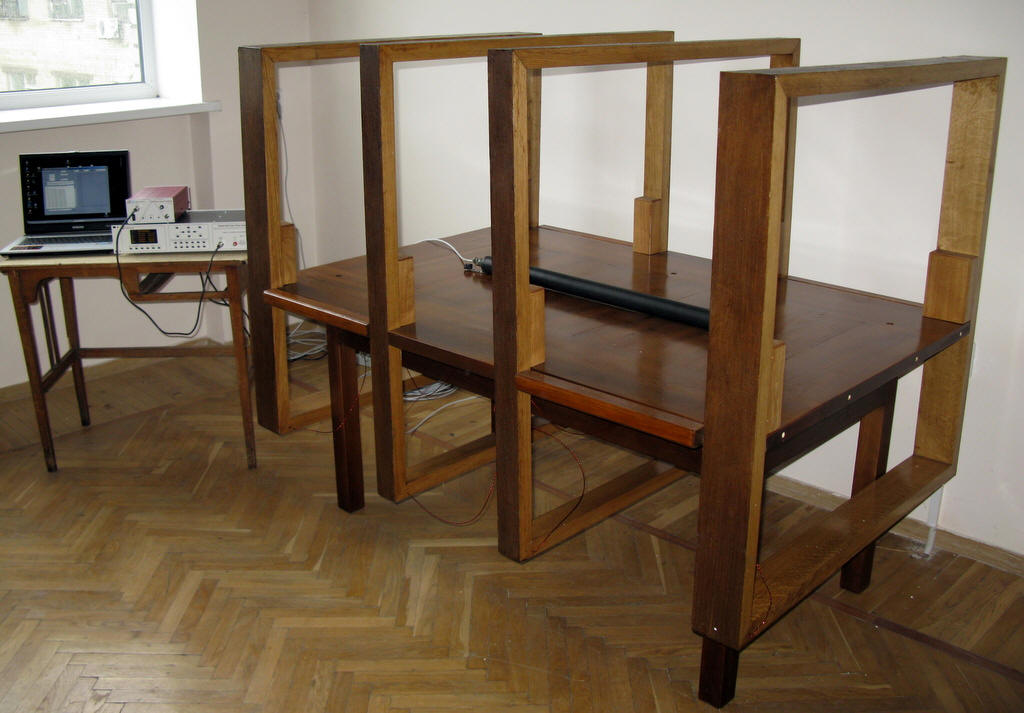Fig. 1. Calibrating Coil System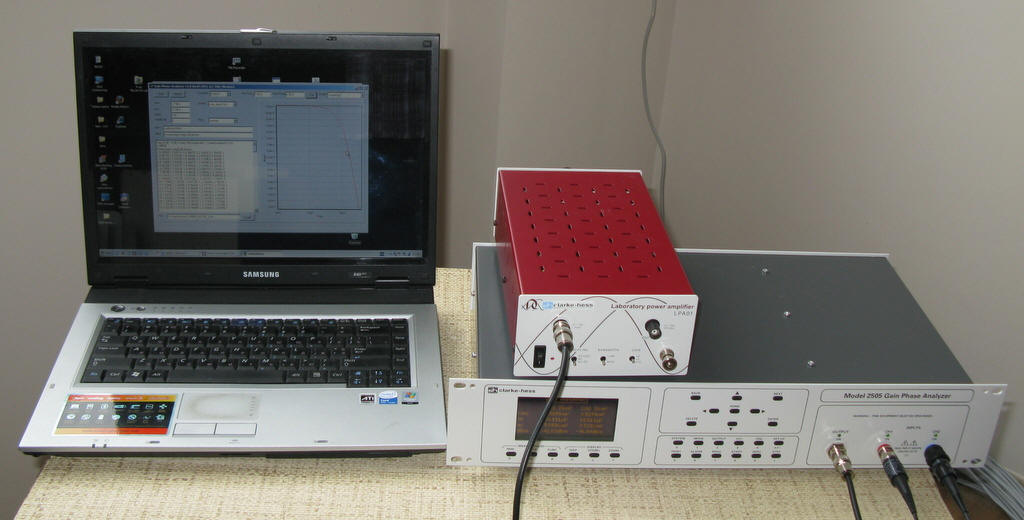Fig. 2. Clarke-Hess measuring instrument with amplifier LPA01 and control notebook

The coils’ configuration is shown in Fig. 3. This configuration was calculated and optimized by computer simulation for provision of maximal compactness at given maximum magnetic field nonuniformity (in given cylindrical volume) and upper limit of frequency range.

The calculated coils’ parameters are shown in Table 1. The maximal calibrating coils’ nonuniformity on cylindrical surface of radius R=0.05 m and 0.1 m against distance from coil system centre along coils axis is shown in Fig. 4. From this plot it is clearly seen that CCS provides magnetic field sensor calibration in cylindrical volume of diameter 0.2 m and length 1.2 m with nonuniformity less than 0.01 (1%). For sensor with length less than 30 cm, this nonuniformity is less than 0.1%.

The measured CCS constant K, after coil assembling, is 15.6 nT/mA ± 0.1%.

The magnetic field B in the CCS centre equals

B = I*K,

where I is current in coils.

Note 1. For provision of maximal accuracy (about 0.1%) at current measurements in wide frequency range it is recommended to use reference resistor R with total error less than 0.03%. Thus the current in coils equals

I = U/R,

where U is voltage on reference resistor. For provision of maximal precision the voltage U should be measured with error less than 0.1%. At greater allowable errors it is possible to use for U voltage measurement the data from CHI input 1 (see p. 11-3 of CHI “Instruction Manual”).

Typical electric circuit at magnetic sensor calibrating procedure is shown in Fig. 5.

In upper part of CCs frequency range the correction factor K1 for measured current I is applied

I = K1*U/R,

where

K1 = ((1-(f/f0)2)2+(4*10-9*f)2)-0.5,

f is current frequency, f0 = 2.8*105 Hz is coils resonance frequency. For example, at frequency f = 10 kHz K1 = 1.0006, however at f = 200 kHz K1 = 1.4289.

Note 2. At DC measurements a “+” clamp of DC power supply should be connected to red clamp of calibrating coils. A “-” clamp of DC power supply should be connected to black clamp of calibrating coils via reference resistor R. In this case magnetic field vector B will be oriented along coil axis from table side with input clamps to opposite table side.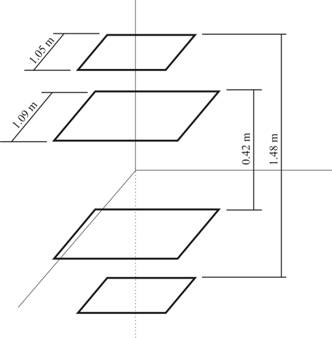Fig. 3. The coils’ configuration

Note 3. In AC mode maximal current in coils is limited by the maximal current of LPA01 (0.75 A, peak value, see LPA01 brochure). In DC mode the maximal current is limited by dissipated heat in winding and time. As a current source in DC mode any stable and low noisy power supply can be used. The DC value in calibrating coils I and time of continuous work in DC operation mode T can be described by simple equation

T = T0*(I0/I)2,

where T0 = 1 hour, I0 = 1 A, I ≤ 4 A.

Table 1. Calculated coils’ parameters

 Coil parameter Value Inner coils’ side length, m 1.09 Outer coils’ side length, m 1.05 Distance between inner coils, m 0.42 Distance between outer coils, m 1.48 Number of turns in inner coils 6 Number of turns in outer coils 10 Wire diameter, mm 1.1 Coils total DC resistance, Ohm 2 Winding pitch (at one turn), mm 20 Coils constant (calculated), nT/mA 15.581 Coils constant (measured), nT/mA 15.6±0.1% Coils resonance frequency, kHz 280 Coils frequency range, kHz 0 - 200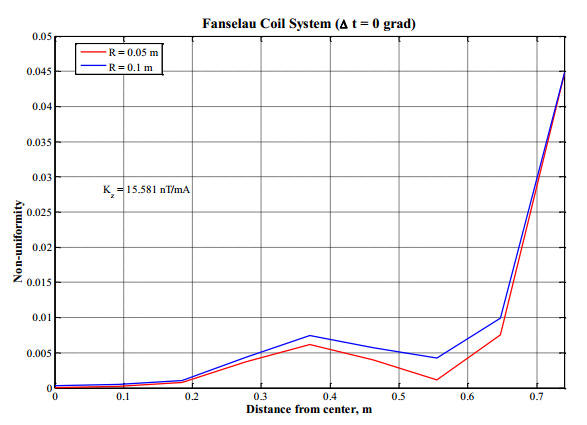Fig. 4. Maximal magnetic field nonuniformity on cylindrical surface of radius R=0.05 m and 0.1 m against distance from coil system centre along coils axis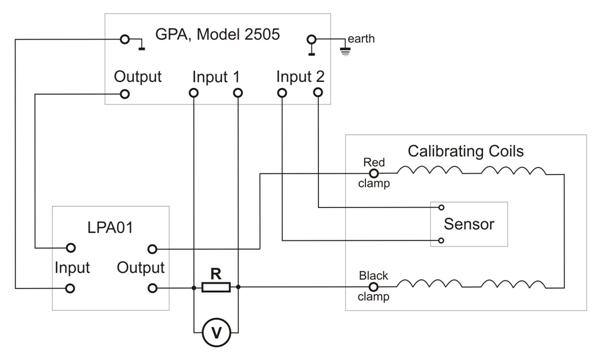Fig. 5. Typical electric circuit at magnetic sensor calibrating procedure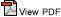Copyright © ISR '2001-2013